# Multinomial distribution

(diff) ← Older revision | Latest revision (diff) | Newer revision → (diff)

polynomial distribution

The joint distribution of random variables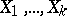that is defined for any set of non-negative integerssatisfying the condition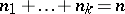,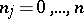,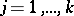, by the formula(*)

where(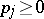,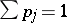) are the parameters of the distribution. A multinomial distribution is a multivariate discrete distribution, namely the distribution for the random vector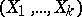with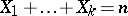(this distribution is in essence-dimensional, since it is degenerate in the Euclidean space ofdimensions). A multinomial distribution is a natural generalization of a binomial distribution and coincides with the latter for. The name of the distribution is given because the probability (*) is the general term in the expansion of the multinomial. The multinomial distribution appears in the following probability scheme. Each of the random variablesis the number of occurrences of one of the mutually exclusive events,, in repeated independent trials. If in each trial the probability of event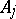is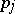,, then the probability (*) is equal to the probability that intrials the eventswill appear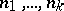times, respectively. Each of the random variables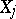has a binomial distribution with mathematical expectation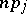and variance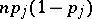.

The random vectorhas mathematical expectation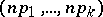and covariance matrix, where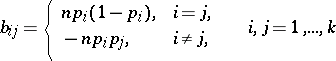(the rank of the matrixisbecause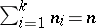). The characteristic function of a multinomial distribution is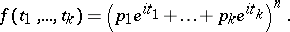For, the distribution of the vectorwith normalized components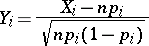tends to a certain multivariate normal distribution, while the distribution of the sum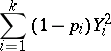(which is used in mathematical statistics to construct the "chi-squared" test) tends to the "chi-squared" distribution withdegrees of freedom.

How to Cite This Entry:
Multinomial distribution. Encyclopedia of Mathematics. URL: http://encyclopediaofmath.org/index.php?title=Multinomial_distribution&oldid=11687
This article was adapted from an original article by A.V. Prokhorov (originator), which appeared in Encyclopedia of Mathematics - ISBN 1402006098. See original article